# SQL Comparison Operators (Equal, Not Equal, Less than, Grater than)

In SQL, the comparison operators are useful to compare one expression with another expression using mathematical operators like equal (=), greater than (>), less than (*), greater than or equal to (>=), less than or equal to (<=), not equal (<>), etc. on SQL statements. In SQL, we have different types of comparison operators available those are

• SQL Equal (=) Operator
• SQL Not Equal (!= or <>) Operator
• SQL Greater Than (>) Operator
• SQL Less Than (<) Operator
• SQL Greater Than or Equal To (>=) Operator
• SQL Less Than or Equal To (<=) Operator
• SQL Not Less Than (!<) Operator
• SQL Not Greater Than (!>) Operator

Before we check each operator, try to create an “EmployeeDetails” table by using the below script in the SQL database.

create table EmployeeDetails(empid int, empname varchar(50),designation varchar(50),salary int,Location varchar(50))

insert into EmployeeDetails
values(1,'suresh','software engineer',25000,'chennai'),
(2,'rohini','AEO',15000,'chennai'),
(4,'mahendra','CA',75000,'guntur'),
(5,'sateesh','Doctor',65000,'guntur')

select * from EmployeeDetails

Once we run the above SQL script, our table “EmployeeDetails” will create, and the result as shown below.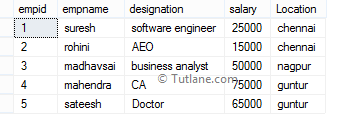Now we will learn each comparison operator in SQL with proper examples.

## SQL Equal (=) Operator

In SQL, the equal operator is useful to check whether the given two expressions are equal or not. If it’s equal, then the condition will be true, returning matched records.

Example:

If we run the following SQL statement for the equal operator, it will return records where empid equals 1.

SELECT * FROM EmployeeDetails WHERE empid = 1

When we execute the above SQL equal operator query, we will get the result below.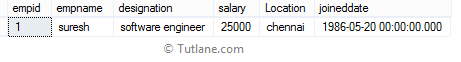## SQL Not Equal (!=) Operator

In SQL, the not equal operator is used to check whether two expressions are equal or not. If it’s not equal, then the condition will be true, and it will return not matched records.

Example:

If we run the following SQL statement for not equal operator it will return records where empid is not equal to 1.

SELECT * FROM EmployeeDetails WHERE empid != 1

When we execute the above SQL not equal operator query, we will get the result below.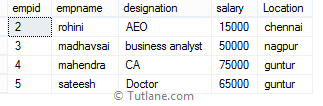## SQL Not Equal (<>) Operator

In SQL, not equal operator is used to check whether two expressions are equal or not. If it’s not equal, then the condition will be true, and it will return not matched records. Both != and <> operators are not equal operators and will return the same result, but the != operator is not an ISO standard.

Example:

If we run the following SQL statement for not equal operator it will return records where empid is not equal to 1.

SELECT * FROM EmployeeDetails WHERE empid <> 1

When we execute the above SQL not equal operator query, we will get the result below.## SQL Greater Than (>) Operator

In SQL, greater than operator is used to check whether the left-hand operator is higher than the right-hand operator or not. If the left-hand operator is higher than the right-hand operator, the condition will be true and return matched records.

Example:

If we run the following SQL statement for greater than operator it will return records where empid greater than 2.

SELECT * FROM EmployeeDetails WHERE empid > 2

When we execute the above SQL greater than the operator query, we will get the result below.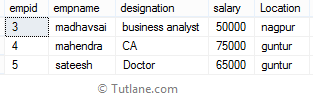## SQL Less Than (<) Operator

In SQL, less than operator is used to check whether the left-hand operator is lower than the right-hand operator or not. If the left-hand operator is lower than the right-hand operator, the condition will be true and return matched records.

Example:

If we run the following SQL statement for less than operator, it will return records where empid is less than 2.

SELECT * FROM EmployeeDetails WHERE empid < 2

When we execute the above SQL less than operator query, we will get the result below.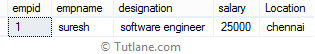## SQL Greater Than or Equal To (>=) Operator

In SQL, greater than or equal to the operator is used to check whether the left-hand operator is higher than or equal to the right-hand operator or not. If the left-hand operator is higher than or equal to the right-hand operator, the condition will be true and return matched records.

Example:

If we run the following SQL statement for greater than or equal to the operator it will return records where empid is higher than or equal to 2.

SELECT * FROM EmployeeDetails WHERE empid >= 2

When we execute the above SQL greater than or equal to operator query, we will get the result below.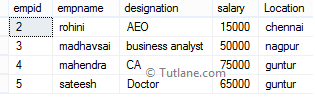## SQL Less Than or Equal To (<=) Operator

In SQL, less than or equal to the operator is useful to check whether the left-hand operator is lower than or equal to the right-hand operator or not. If the left-hand operator is lower than or equal to the right-hand operator, then the condition will be true and return matched records.

Example:

If we run the following SQL statement for less than or equal to the operator, it will return records where empid is lower than or equal to 2.

SELECT * FROM EmployeeDetails WHERE empid <= 2

When we execute the above SQL less than or equal to operator query, we will get the result below.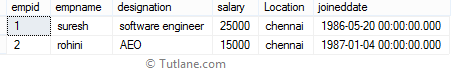## SQL Not Less Than (!<) Operator

In SQL, not less than operator is used to check whether the left-hand operator is not lower than the right-hand operator or not. If the left-hand operator is not lower than the right-hand operator, the condition will be true and return matched records.

Example:

If we run the following SQL statement for not less than operator it will return records where empid is not lower than 2.

SELECT * FROM EmployeeDetails WHERE empid !< 2

When we execute the above SQL not less than operator query, we will get the result below.## SQL Not Greater Than (!>) Operator

In SQL, not greater than operator is used to check whether the left-hand operator is not higher than the right-hand operator or not. If the left-hand operator is not higher than the right-hand operator, the condition will be true and return matched records.

Example:

If we run the following SQL statement for not greater than operator, it will return records where empid is not higher than 2.

SELECT * FROM EmployeeDetails WHERE empid !> 2

When we execute the above SQL not greater than operator query, we will get the result below.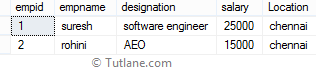For more information related to comparison operators in SQL server, check the following information.

OperatorDescription
Equal (=) Operator The sql equal operator is used to check whether two expressions are equal or not. If it’s equal, the condition will be true and will return matched records.
Not Equal (!=) Operator The sql not equal operator is used to check whether two expressions are equal or not. If it’s not equal, then the condition will be true, and it will return not matched records.
Not Equal (<>) Operator The sql not equal operator is used to check whether two expressions are equal or not. If it’s not equal, then the condition will be true, and it will return not matched records.
Greater Than (>) Operator The sql Greater Than operator is used to check whether the left-hand operator is higher than the right-hand operator or not. If the left-hand operator is higher than the right-hand operator, the condition will be true and return matched records.
Less Than (<) Operator The sql Less Than operator is used to check whether the left-hand operator is lower than the right-hand operator or not. If the left-hand operator is lower than the right-hand operator, the condition will be true and return matched records.
Greater Than or Equal To (>=) Operator The sql Greater Than or Equal To operator is useful to check whether the left-hand operator is higher than or equal to the right-hand operator or not. If the left-hand operator is higher than or equal to the right-hand operator, the condition will be true, returning matched records.
Less Than or Equal To (<=) Operator The sql Less Than or Equal To operator is useful to check whether the left-hand operator is lower than or equal to the right-hand operator or not. If the left-hand operator is lower than or equal to the right-hand operator, the condition will be true and return matched records.
Not Less Than (!<) Operator The sql Not Less Than operator is used to check whether the left-hand operator not lower than the right-hand operator or not. If the left-hand operator is not lower than the right-hand operator, the condition will be true and return matched records.
Not Greater Than(!>) Operator The sql Not Less Than operator is used to check whether the left-hand operator not lower than the right-hand operator or not. If the left-hand operator is not lower than the right-hand operator, the condition will be true and return matched records.